1、三维场景中创建图像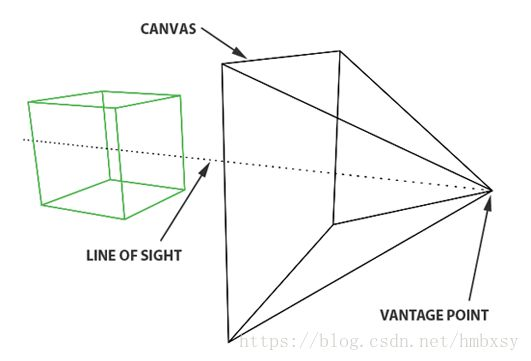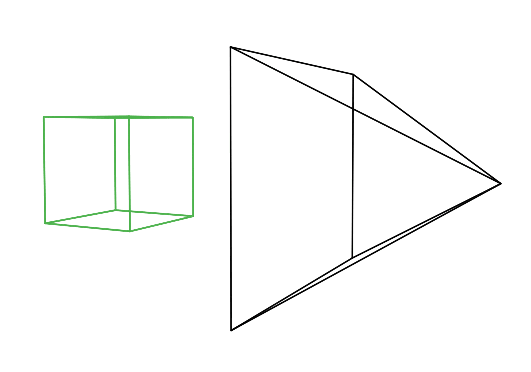2、物体的颜色和亮度

3、光与物体的关系

1、Forward Tracing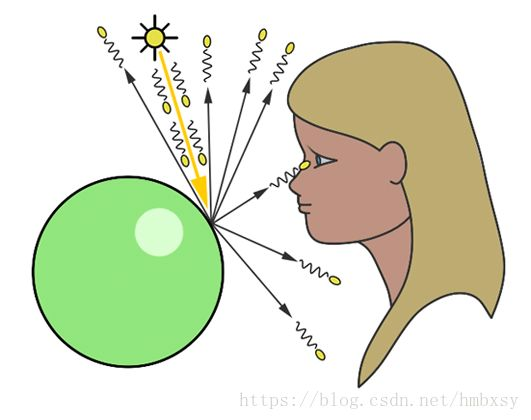Tracing），因为我们是沿着光子从光源向观察者的前进的路径。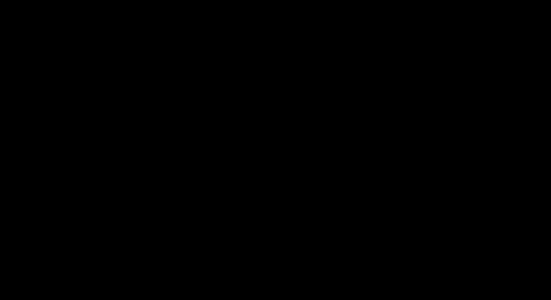2、Backward Tracing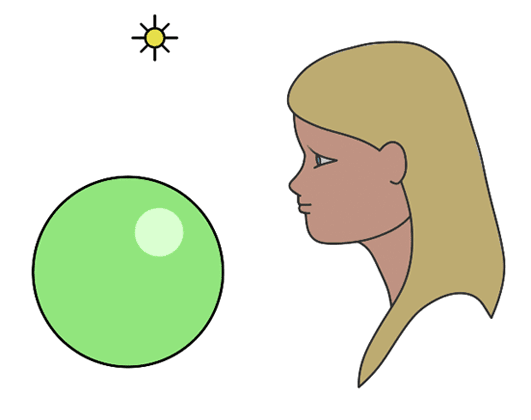1、基本原理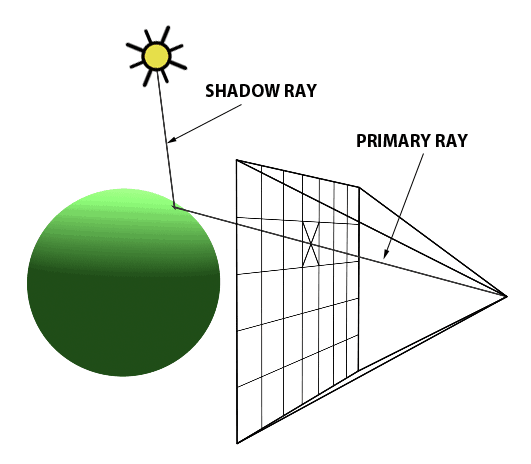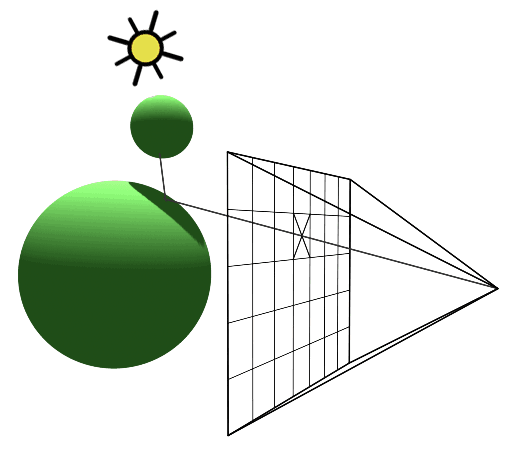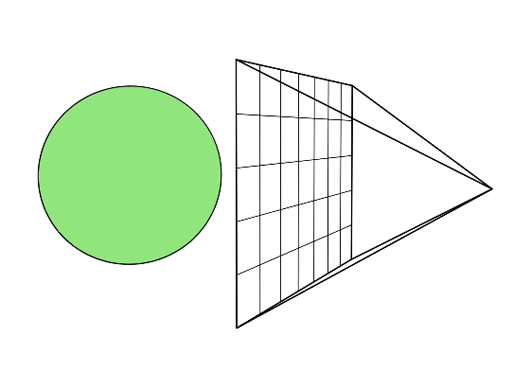2、伪代码

for (int j = 0; j < imageHeight; ++j)
{ for (int i = 0; i < imageWidth; ++i) { // compute primary ray
direction Ray primRay; computePrimRay(i, j, &primRay); // shoot prim ray
in the scene and search for intersection Normal nHit; float minDist =
INFINITY; Object object = NULL; for (int k = 0; k < objects.size();
++k) { if (Intersect(objects[k], primRay, &pHit, &nHit)) { float
distance = Distance(eyePosition, pHit); if (distance < minDistance) {
object = objects[k]; minDistance = distance; // update min distance }
} } if (object != NULL) { // compute illumination Ray shadowRay;
shadowRay.direction = lightPosition – pHit; bool isShadow = false; for
(int k = 0; k < objects.size(); ++k) { if (Intersect(objects[k],
shadowRay)) { isInShadow = true; break; } } } if (!isInShadow)
pixels[i][j] = object->color * light.brightness; else
pixels[i][j] = 0; } }

1、基本原理

• 计算反射

• 计算折射

• 应用菲涅尔方程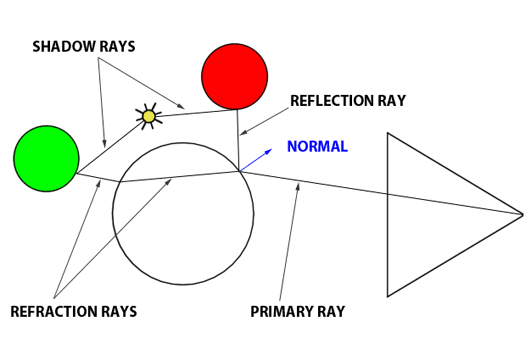2、伪代码

// compute reflection color color
reflectionCol = computeReflectionColor(); // compute refraction color
color refractionCol = computeRefractionColor(); float Kr; // reflection
mix value float Kt; // refraction mix value fresnel(refractiveIndex,
normalHit, primaryRayDirection, &Kr, &Kt); // mix the two color
glassBallColorAtHit = Kr * reflectionColor + (1-Kr) *
refractionColor;